# Electric Field and Potential Questions and Answers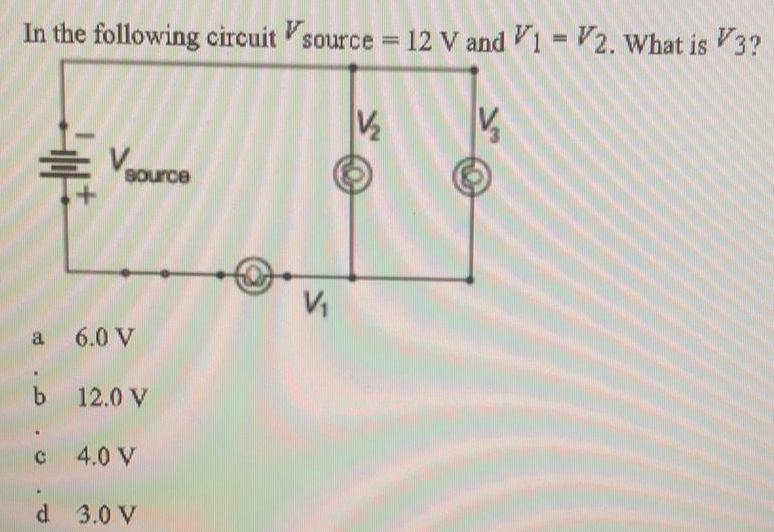Physics
Electric Field and Potential
In the following circuit Vsource = 12 V and V1 V2. What is V3? a 6.0 V b 12.0 V c 4.0 V d 3.0 V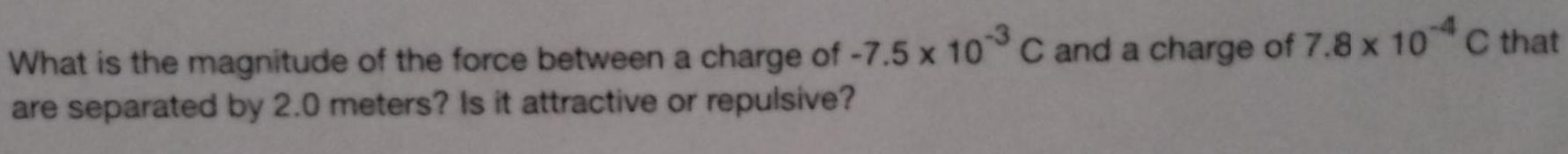Physics
Electric Field and Potential
What is the magnitude of the force between a charge of -7.5 x 10 C and a charge of 7.8 x 10 C that are separated by 2.0 meters? Is it attractive or repulsive?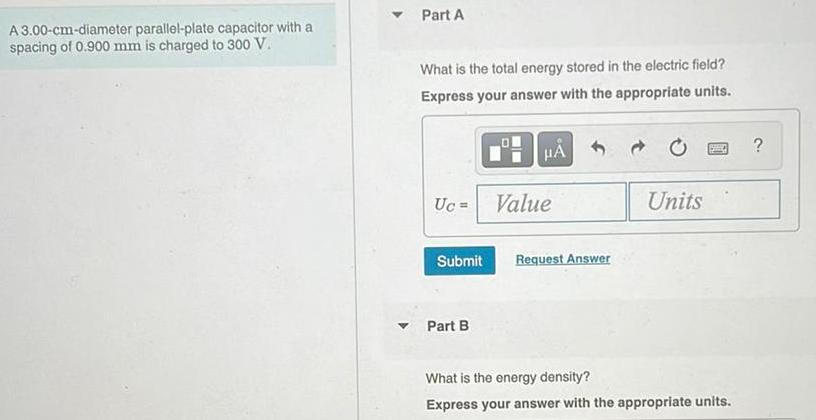Physics
Electric Field and Potential
A 3.00-cm-diameter parallel-plate capacitor with a spacing of 0.900 mm is charged to 300 V. Part A What is the total energy stored in the electric field? Express your answer with the appropriate units. Uc= Part B What is the energy density? Express your answer with the appropriate units.?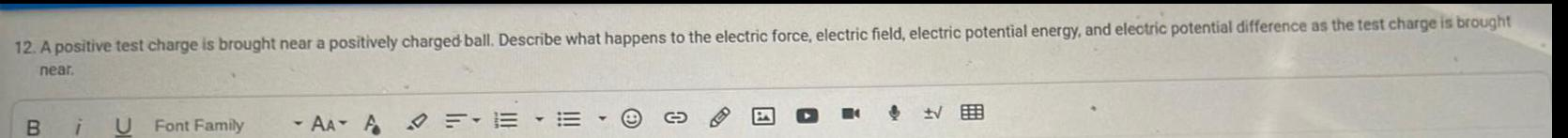Physics
Electric Field and Potential
A positive test charge is brought near a positively charged ball. Describe what happens to the electric force, electric field, electric potential energy, and electric potential difference as the test charge is brought near.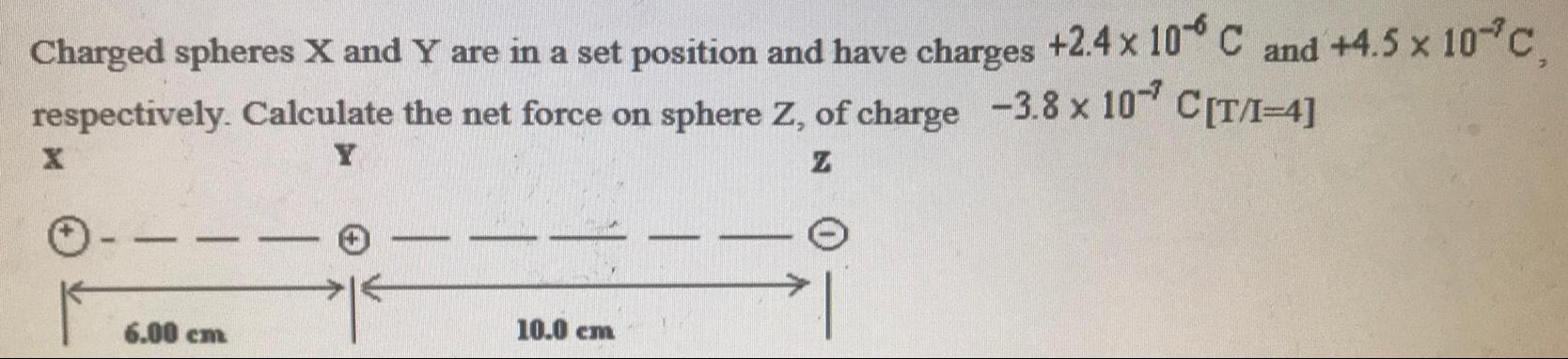Physics
Electric Field and Potential
Charged spheres X and Y are in a set position and have charges +2.4 × 10^-6 C and +4.5 x 10^-7 C respectively. Calculate the net force on sphere Z, of charge -3.8 x 10^-7 C[T/I-4]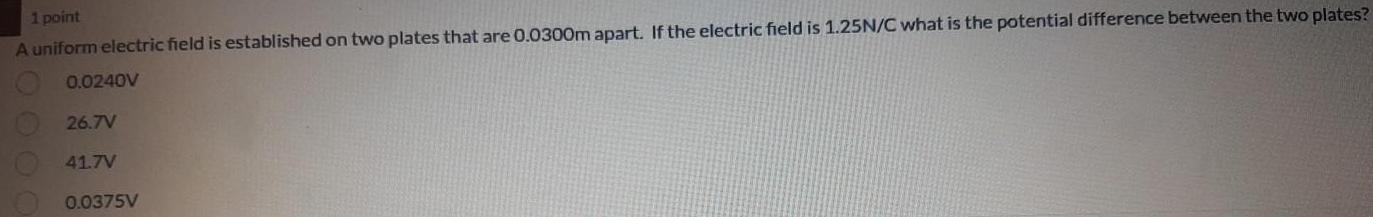Physics
Electric Field and Potential
A uniform electric field is established on two plates that are 0.0300m apart. If the electric field is 1.25N/C what is the potential difference between the two plates? 0.0240V 26.7V 41.7V 0.0375V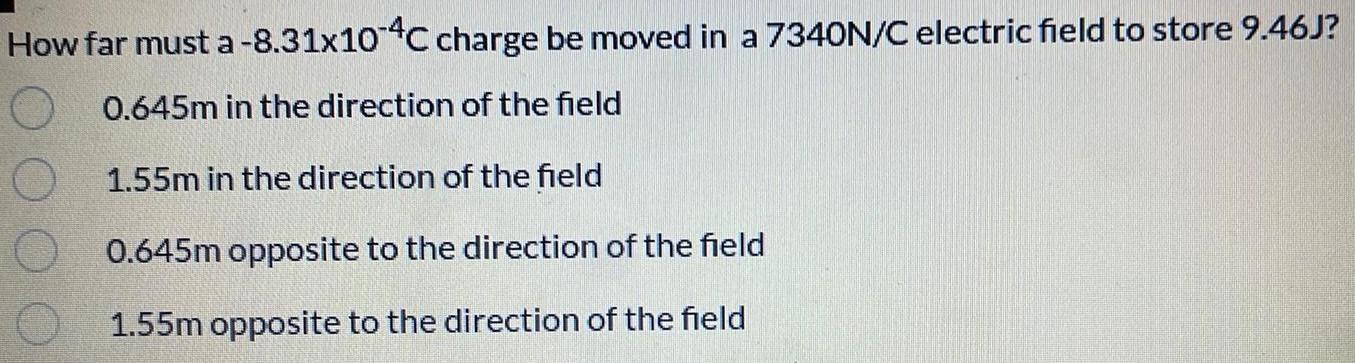Physics
Electric Field and Potential
How far must a-8.31x10 4C charge be moved in a 7340N/C electric field to store 9.46J? 0.645m in the direction of the field 1.55m in the direction of the field 0.645m opposite to the direction of the field 1.55m opposite to the direction of the field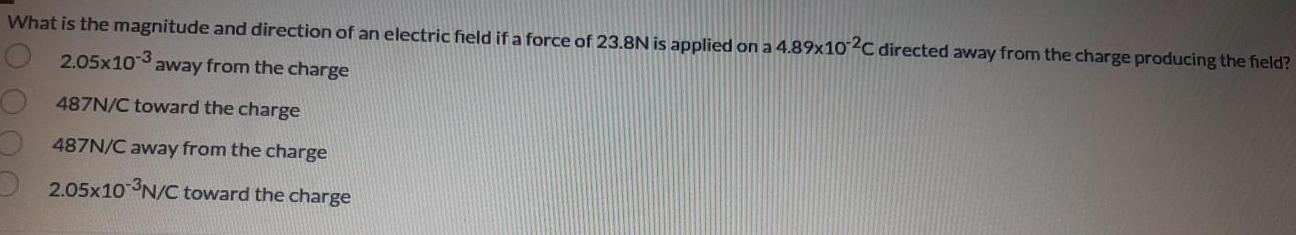Physics
Electric Field and Potential
What is the magnitude and direction of an electric field if a force of 23.8N is applied on a 4.89x102C directed away from the charge producing the field? 2.05x103 away from the charge 487N/C toward the charge 487N/C away from the charge 2.05x10 ³N/C toward the charge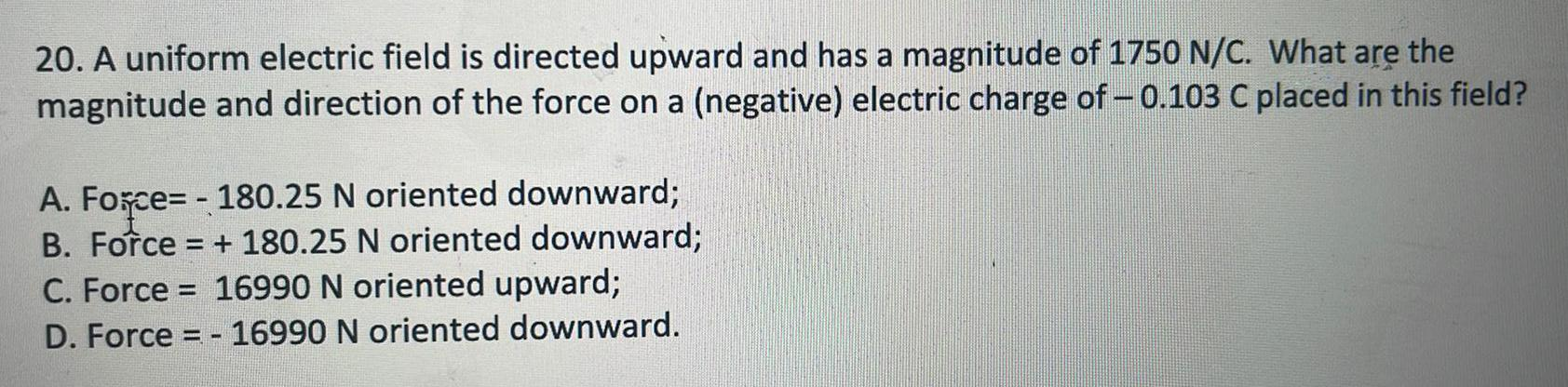Physics
Electric Field and Potential
A uniform electric field is directed upward and has a magnitude of 1750 N/C. What are the magnitude and direction of the force on a (negative) electric charge of – 0.103 C placed in this field? A. Force= - 180.25 N oriented downward; B. Force = + 180.25 N oriented downward; C. Force = 16990 N oriented upward; D. Force = - 16990 N oriented downward.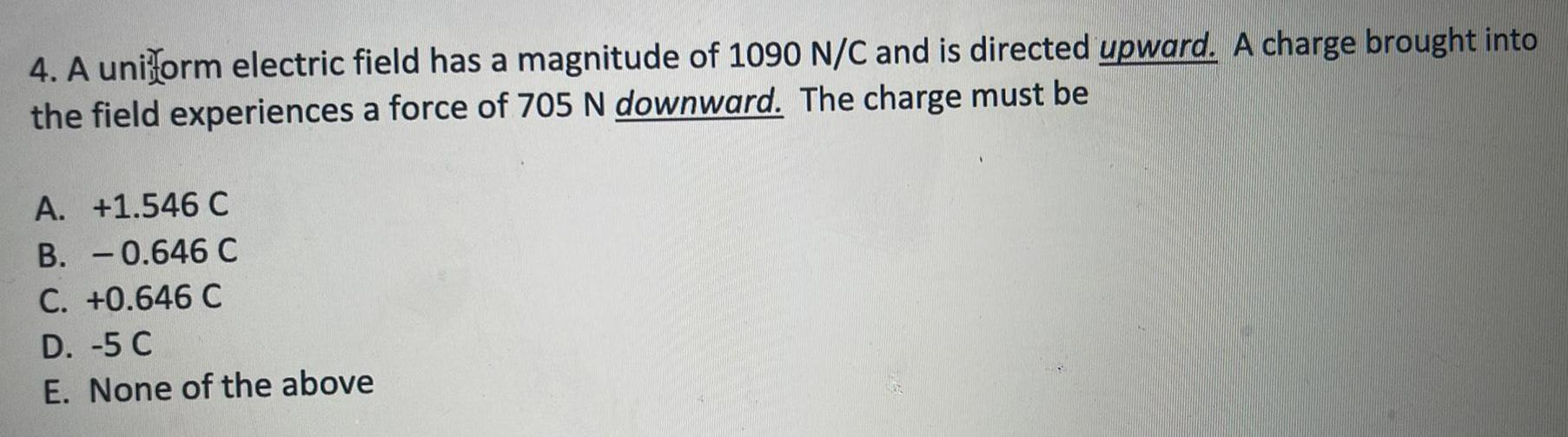Physics
Electric Field and Potential
A uniform electric field has a magnitude of 1090 N/C and is directed upward. A charge brought into the field experiences a force of 705 N downward. The charge must be A. +1.546 C B. -0.646 C C. +0.646 C D. -5 C E. None of the above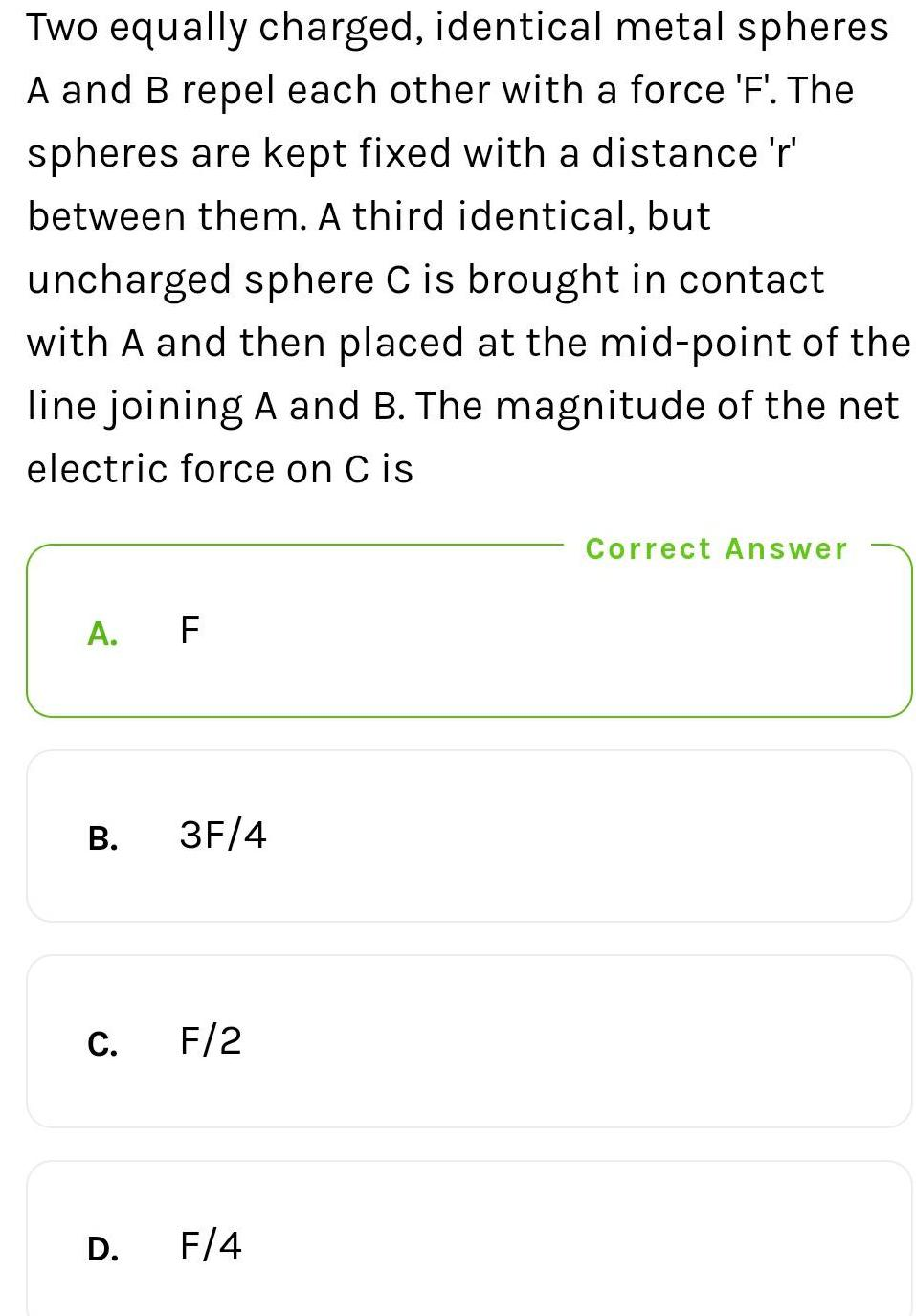Physics
Electric Field and Potential
Two equally charged, identical metal spheres A and B repel each other with a force 'F'. The spheres are kept fixed with a distance 'r' between them. A third identical, but uncharged sphere C is brought in contact with A and then placed at the mid-point of the line joining A and B. The magnitude of the net electric force on C is A. F B. 3F/4 C. F/2 D. F/4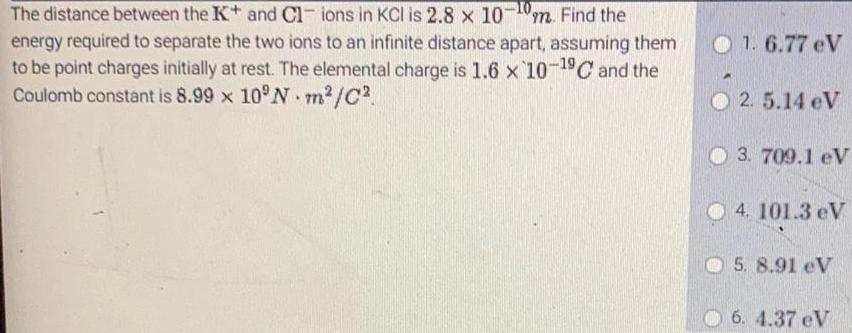Physics
Electric Field and Potential
The distance between the K+ and Cl- ions in KCl is 2.8 x 10^-10. Find the energy required to separate the two ions to an infinite distance apart, assuming them to be point charges initially at rest. The elemental charge is 1.6 x 10^-19C and the Coulomb constant is 8.99 x 10^9N m²/C² 1. 6.77 eV 2. 5.14 eV 3. 709.1 eV 4. 101.3 eV 5. 8.91 eV 6. 4.37 eV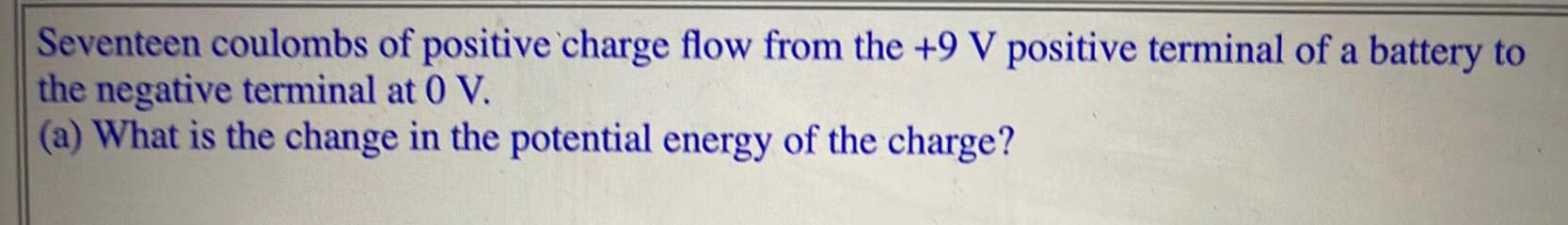Physics
Electric Field and Potential
Seventeen coulombs of positive charge flow from the +9 V positive terminal of a battery to the negative terminal at 0 V. (a) What is the change in the potential energy of the charge?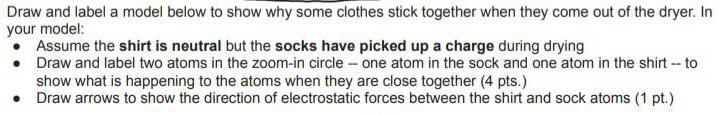Physics
Electric Field and Potential
Draw and label a model below to show why some clothes stick together when they come out of the dryer. In your model: Assume the shirt is neutral but the socks have picked up a charge during drying Draw and label two atoms in the zoom-in circle - one atom in the sock and one atom in the shirt -- to show what is happening to the atoms when they are close together (4 pts.) Draw arrows to show the direction of electrostatic forces between the shirt and sock atoms (1 pt.)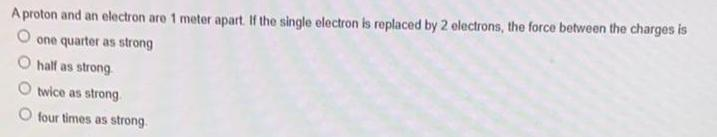Physics
Electric Field and Potential
A proton and an electron are 1 meter apart. If the single electron is replaced by 2 electrons, the force between the charges is one quarter as strong half as strong twice as strong. four times as strong.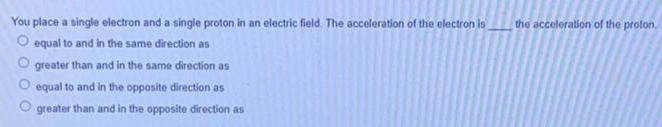Physics
Electric Field and Potential
You place a single electron and a single proton in an electric field. The acceleration of the electron is the acceleration of the proton. equal to and in the same direction as greater than and in the same direction as equal to and in the opposite direction as greater than and in the opposite direction as